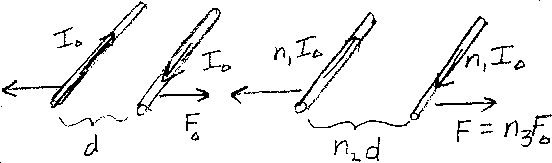Problem C13: Initially two current carrying straight wires that are infinite in length and parallel have a current of magnitude I0 in each wire (see left diagram). The wires are separated by a distance d, and the magnitude of the repulsive force that the wires feel is F0. Then the magnitude of the current in each wire is changed to n1I0, and the wires are separated by a distance n2d (see right diagram). If the magnitude of the force between the two wires is now F=n3F0, what is n3?n1 = n2 = Input n3:
If you are currently in my class, you can record your grade by entering your name and student ID number (without the leading zeros) below and clicking on "record grade".
 First Name = Last Name = ID = Problem: